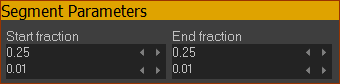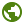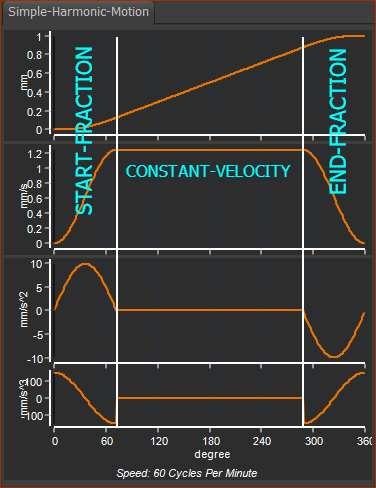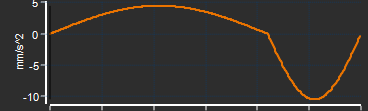﻿ MotionDesigner Reference & User Interface > Motion Laws > Ramp Motion-Law

# Ramp Motion-Law

Navigation:  MotionDesigner Reference & User Interface > Motion Laws >

# Ramp Motion-Law

### Ramp Cam-Law, Motion-Law

#### The Ramp Motion-Law has three phases:

 Phase 1: Acceleration : first ½ wave of a Sine function Phase 2: Zero Acceleration : Constant Velocity Phase 3: Deceleration : last ½ wave of a Sine function : 25% of the Segment Width

#### Segment EditorandBlend-Point Editor - Motion-Values

You CAN specify the:

 •Start-Position The Start-Position usually flows from the End-Position of the •End-Position

You CANNOT specify the:

 •Start-Velocity, End-Velocity •Start-Acceleration, End-Acceleration •Start-Jerk, End-Jerk

Segment ParametersStart-Fraction × 100 = % of Segment-Width to accelerate from zero-velocity* to maximum-velocity End-Fraction × 100 = % of Segment-Width to decelerate from maximum-velocity to zero-velocity**. Start-Fraction + End Fraction 1 Constant-Velocity % = 1- Start-Fraction – End-Fraction * assuming Start-Range is zero ** assuming End-Range is zero

Segment-Range

 Start-Range - remove the start of the Ramp Motion-Law End- Range- remove the end of the Ramp Motion-Law 0 ≤ Start-Range < End-Range ≤ 1

See also :Tutorial 5: Edit the Start of a Traditional Motion-Law

See also :Tutorial 9: Asymmetrical Motions1 x Ramp Segment, Symmetrical

Applications

Asymmetric Segments, in which the Acceleration is longer or shorter than the Deceleration.

Motion with a long displacement, in which the maximum-velocity is less than other motion-laws.

EXAMPLE : see image below.

In the example:

Start-Fraction ≠ End-Fraction

Start-Fraction = 0.7

End-Fraction = 0.3

Start-Fraction + End-Fraction = 1

Constant-Velocity % = 0%E.g.: RAMP Segment: Start-Fraction = 0.7, End-Fraction = 0.3
(Constant-Velocity Fraction = 0)